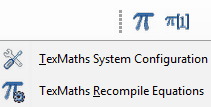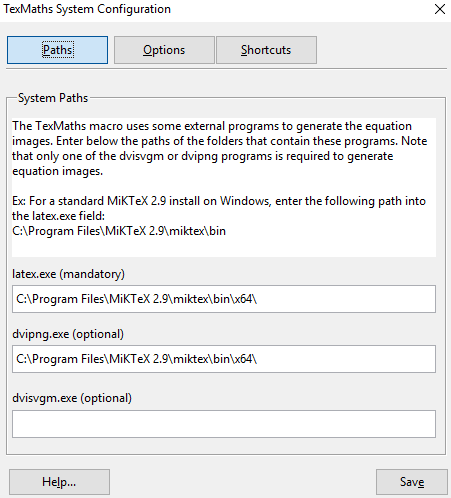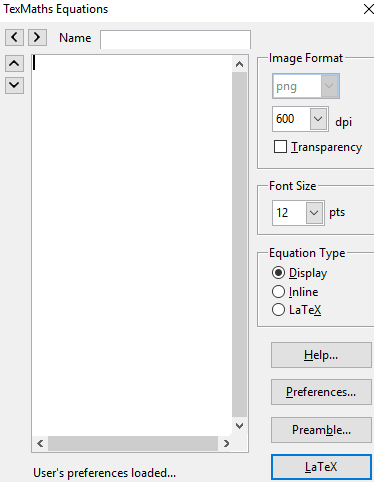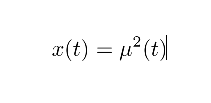Editor Ratings:
User Ratings:
[Total: 0 Average: 0]

In this tutorial, I will explain how to add maths equations in LibreOffice Writer. The method explained in this tutorial will also work for LibreOffice Impress and Draw.

To insert maths equations in LibreOffice, I am going to use TexMaths, which is a free Latex equation editor for LibreOffice. This LibreOffice Maths extension will provide you an interface in which you can specify complex Maths equations to insert in LibreOffice. This extension also required free software MikTex to be installed. So, you’ll need to install that first.If you are looking for a Tex editor for Windows, then you can go with EmilTex editor which comes with preview support, syntax highlighting, etc. We have also covered an MS Word add-in to solve equations and plot graphs.

## How to insert Maths equations in LibreOffice Writer, Impress, and Draw:

Step 1: You first have to download MikTex and install it. After that, download TexMaths LibreOffice extension from the link at the end of this tutorial and install it. To install it, open LibreOffice extension manager by pressing Control+Alt+E hotkey and then click on Add button. After that, browse the path of TexMaths extension and it will be then automatically installed.

Step 2: After TexMaths extension is installed in LibreOffice, you will see its icons on LibreOffice toolbar. Before using this LibreOffice extension, you have to first configure it. From TexMaths toolbar, select the Texmaths System Configuration button to bring forth its configuration window.Then specify the path of latex.exe. This file gets installed with MikTex, you can find it in C:\Program Files\MiKTex x.x\miktex\bin (default). After that, you have to specify the path of dvipng.exe, you can find it in the same folder as latex.exe. At last, click on the Save button to save your preferences. If everything works fine, you won’t see any errors and the configuration window will close smoothly.Step 3: To insert maths equation in LibreOffice, click on the TexMaths Equation button (Pi symbol) and then specify the math equation using Latex. You can also set the font size of the equation and set image resolution (in dpi). After specifying the math equation, click on the LaTex button to generate the image of the math equation and insert it in LibreOffice Writer. You can also follow this step to insert math equations as images in LibreOffice Impress and Draw.After you will click on the Latex button, the maths equation will be inserted in LibreOffice Writer, as shown in the screenshot below.## Verdict:

In this tutorial, I have explained how to add maths equations in LibreOffice. You can use this method to insert maths equations as images in LibreOffice by specifying maths equation.